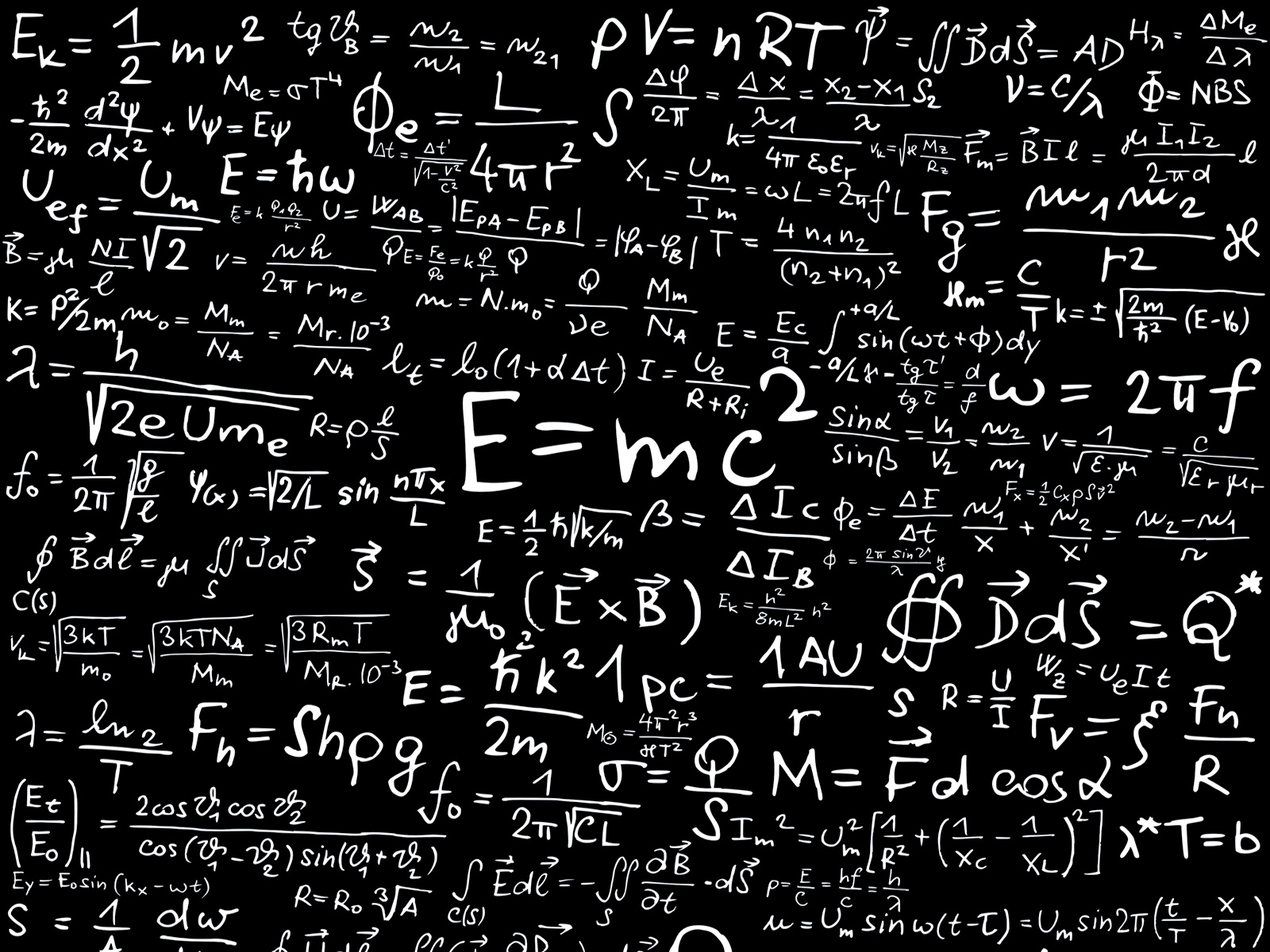# Useful Formulas

What is a problem? A problem = a fact + a judgment. That is a simple formula that tells us something about the way the world works. Maths is full of formulas, and that can intimidate some people if they don’t understand them or can’t remember the right one to use.

However, formulas should be our friends, as they help us to do sometimes complex calculations accurately and repeatably in a consistent and straightforward way. The following is a list of the most useful ones I’ve come across while teaching Maths to a variety of students at a variety of ages and at a variety of stages in their education.

## Averages

• The mean is found by adding up all the values and dividing the total by how many there are, eg the mean of the numbers 1-10 is 5.5, as 1 + 2 + 3 + 4 + 5 + 6 + 7 + 8 + 9 + 10 = 55, and 55 ÷ 10 = 5.5.
• The mode is the most common value (or values), eg the mode of 1, 2, 2, 3, 4, 5 is 2.
• The median of an odd number of values sorted by size is the one in the middle, eg the median of the numbers 1-5 is 3. The median of an even number of values is the mean of the two numbers in the middle, eg the median of the numbers 1-10 is 5.5, as 5 and 6 are the numbers in the middle, and 11 ÷ 2 = 5.5.
• The range is the highest value minus the lowest, eg the range of the numbers 1-10 = 10 – 1 = 9.

## Geometry

• Angles around a point add up to 360º
• Angles on a straight line add up to 180º
• Opposite angles are equal, ie the two pairs of angles opposite each other when two straight lines bisect (or cross) each other
• Alternate angles are equal, ie the angles under the arms of a ‘Z’ formed by a line (or ‘transversal’) bisecting two parallel lines
• Corresponding angles are equal, ie the angles under the arms of an ‘F’ formed by a line (or ‘transversal’) bisecting two parallel lines
• Complementary angles add up to 90º
• Any straight line can be drawn using y = mx + c, where m is the gradient and c is the point where the line crosses the y-axis (the ‘y-intercept’)
• The gradient of a straight line is shown by δy/δx (ie the difference in the y-values divided by the difference in the x-values of any two points on the line, usually found by drawing a triangle underneath it)

## Polygons

• Number of diagonals in a polygon = (n-3)(n÷2) where n is the number of sides
• The sum of the internal angles of a polygon = (n-2)180º, where n is the number of sides
• Any internal angle of a regular polygon = (n-2)180º ÷ n, where n is the number of sides

## Rectangles

• Perimeter of a rectangle = 2(l + w), where l = length and w = width
Note that this is the same formula for the perimeter of an L-shape, too.
• Area of a rectangle = lw, where l = length and w = width

## Trapeziums

• Area of a trapezium = lw, where l = average length and w = width (in other words, you have to add both lengths together and divide by two in order to find the average length)

## Triangles (Trigonometry)

• Area of a triangle = ½bh, where b = base and h = height
• Angles in a triangle add up to 180º
• Pythagoras’s Theorem: in a right-angled triangle, a² + b² = c², ie the area of a square on the hypotenuse (or longest side) is equal to the sum of the areas of squares on the other two sidesCircles

• Circumference of a circle = 2πr, where r = radius
• Area of a circle = πr², where r = radius
• π = 3.14 to two decimal places and is sometimes given as 22/7

## Spheres

• Volume of a sphere = 4/3πr³, where r = radius
• Surface area of a sphere = 4πr², where r = radius

## Cuboids

• Volume of a cuboid = lwh, where l is length, w is width and h is height
• Surface area of a cuboid = 2(lw + lh + wh), where l is length, w is width and h is height

## Number Sequences

• An arithmetic sequence (with regular intervals) = xn ± k, where x is the interval (or difference) between the values, n is the value’s place in the sequence and k is a constant that is added or subtracted to make sure the sequence starts at the right number, eg the formula for 5, 8, 11, 14…etc is 3n + 2
• The sum of n consecutive numbers is n(n + 1)/2, eg the sum of the numbers 1-10 is 10(10 + 1)/2 = 110/2 = 55

## Other

• Speed = distance ÷ time
• Profit = sales – cost of goods sold
• Profit margin = profit ÷ sales
• Mark-up = profit ÷ cost of goods sold